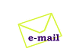ISE 5.2からISE 6.2へ移行し、回路が起動しなくなってしまいましたので、対策しました。

2004/05/25-

クロックを適当にカウントして、リセット信号を出力しています。

 （１）リセット発生回路例 ```library IEEE; use IEEE.std_logic_1164.all; entity ARST is port ( CLK: in STD_LOGIC; RSTO: out STD_LOGIC ); end ARST; architecture ARST_arch of ARST is signal CNTDYN: INTEGER range 0 to 255; begin process (CLK) begin if(CLK = '1' and CLK'event) then if(CNTDYN = 128) then RSTO <= '0'; else CNTDYN <= CNTDYN + 1; RSTO <= '1'; end if; end if; end process; end ARST_arch; ```メールでのご質問はこちらへどうぞ